# Order formulas for symplectic groups

This article describes order formulas for the symplectic group of finite degree over a finite field and its variants.

## For a finite field of size$q$

### Formulas

In the formulas below, the field size is$q$ and the degree (order of matrices involved, dimension of vector space being acted upon) is$n = 2m$. It's easier to write the formulas in terms of$m$ rather than$n$. The characteristic of the field is a prime number$p$.$q$ is a prime power with underlying prime$p$. We let$r = \log_pq$, so$q = p^r$ and$r$ is a nonnegative integer.

In the table below,$\Phi_d(q)$ stands for the$d^{th}$ cyclotomic polynomial evaluated at$q$.

Group Symbolic notation Order formula Order formula (powers of$q$ taken out) Order formula (maximally factorized) Degree as polynomial in$q$ (same as algebraic dimension) Multiplicity of factor$q$ (note$m = n/2$ Multiplicity of factor$q - 1$ Quick explanation for order
symplectic group$Sp(2m,q) = Sp(n,q)$ or$Sp(2m,\mathbb{F}_q) = Sp(n,\mathbb{F}_q)$$\prod_{i=1}^m [(q^{2i} - 1)q^{2i-1}]$$q^{m^2} \prod_{i=1}^m (q^{2i} - 1)$$q^{m^2} \prod_{d=1}^m \Phi_d(q^2)^{\lfloor m/d \rfloor}$$m^2 = n^2/4$$m = n/2$ See full explanation below.
projective symplectic group$PSp(2m,q) = PSp(n,q)$ or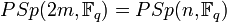$PSp(2m,\mathbb{F}_q) = PSp(n,\mathbb{F}_q)$$\frac{\prod_{i=1}^m [(q^{2i} - 1)q^{2i-1}]}{\operatorname{gcd}(2,q-1)}$$q^{m^2} \frac{\prod_{i=1}^m (q^{2i} - 1)}{\operatorname{gcd}(2,q-1)}$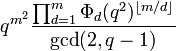$q^{m^2} \frac{\prod_{d=1}^m \Phi_d(q^2)^{\lfloor m/d \rfloor}}{\operatorname{gcd}(2,q-1)}$$m^2 = n^2/4$$m = n/2$ See full explanation below.

### Explanation for order of symplectic group

We describe here the reasoning behind the formula for the order of the general linear group$Sp(2m,q) = Sp(2m,\mathbb{F}_q)$.

The order equals the number of choices of basis for$\mathbb{F}_q^{2m}$ where the basis is an ordered symplectic basis: the basis comes in the form of an ordered collection of$m$ ordered pairs. The conditions are as follows: with respect to the original symplectic form, the two-dimensional subspaces spanned by these are mutually orthogonal, and the form applied to the first and second vector within each pair gives the value 1.

The order of the symplectic group is the number of possible pairs of this sort.

For the first vector (say$v_1$) of the first pair, there are$q^{2m} - 1$ choices. Let's say$w_1$ is the second vector of the first pair. The bilinear form gives a linear functional$x \mapsto b(v_1,x)$ which is nonzero. We want$w_1$ to be in the pre-image of 1 under this functional. The pre-image of any field element under a linear functional is an affine subspace of codimension one, which means there are$q^{2m-1}$ possibilities. The number of possibilities for the first pair are thus$(q^{2m} - 1)(q^{2m - 1})$.

Once the first pair is chosen, all the remaining pairs must be chosen from the$(2m - 2)$-dimensional subspace orthogonal to its span, and within that space, everything proceeds exactly the same way as if w were solving the original problem for$2(m - 1)$ instead of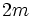$2m$. In other words, we have: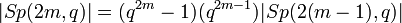$|Sp(2m,q)| = (q^{2m} - 1)(q^{2m - 1})|Sp(2(m-1),q)|$

Carrying through the induction, and noting that$|Sp(0,q)| = 1$, we have:$|Sp(2m,q)| = \prod_{i=1}^m [(q^{2i} - 1)(q^{2i - 1})]$

Collecting the powers of$q$, we note that the exponents are$1,3,5,\dots,2m-1$. They add up to$m^2$, because$1 + 3 + 5 + \dots + (2m - 1) = m^2$, so we get:$|Sp(2m,q)| = q^{m^2} \prod_{i=1}^m (q^{2i} - 1)$

### Explanation for order of projective symplectic group

The center of the symplectic group is trivial in characteristic two, and isomorphic to cyclic group:Z2 (the 1 and -1 scalar matrices) otherwise. The order is thus equal to that of the symplectic group in characteristic two, and half the order of the symplectic group otherwise. A simply way of writing this is:$|PSp(2m,q)| = \frac{|Sp(2m,q)|}{\operatorname{gcd}(2,q-1)} = \frac{q^{m^2} \prod_{i=1}^m (q^{2i} - 1)}{\operatorname{gcd}(2,q-1)}$

### Particular cases$m$$2m$ Order formula for$Sp(2m,q)$
1 2$q(q^2 - 1) = q^3 - q$
2 4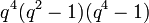$q^4(q^2 - 1)(q^4 - 1)$
3 6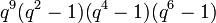$q^9(q^2 - 1)(q^4 - 1)(q^6 - 1)$